Electron. J. Differential Equations, Vol. 2017 (2017), No. 19, pp. 1-15.

### Semiclassical solutions of perturbed biharmonic equations with critical nonlinearity Yubo He, Xianhua Tang, Wen Zhang

Abstract:
We consider the perturbed biharmonic equations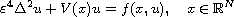and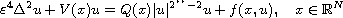where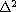is the biharmonic operator,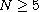,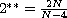is the Sobolev critical exponent, Q(x) is a bounded positive function. Under some mild conditions on V and f, we show that the above equations have at least one nontrivial solution provided that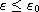, where the bound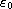is formulated in terms of N, V, Q and f.

Submitted November 15, 2016. Published January 16, 2017.
Math Subject Classifications: 35J35, 35J60, 58E05, 58E50.
Key Words: Perturbed biharmonic equation; semiclassical solution; critical nonlinearity.

Show me the PDF file (279 KB), TEX file for this article.Yubo He School of Mathematics and Statistics Central South University Changsha, 410083 Hunan, China email: hybmath@163.com Xianhua Tang School of Mathematics and Statistics Central South University Changsha, 410083 Hunan, China email: tangxh@mail.csu.edu.cn Wen Zhang School of Mathematics and Statistics Hunan University of Commerce Changsha, 410205 Hunan, China email: zwmath2011@163.com